# A company is considering whether to buy a new machine

• Homework Help
• 62
• 100% (5) 5 out of 5 people found this document helpful

This preview shows page 8 - 10 out of 62 pages.

9. A company is considering whether to buy a new machine, which costs \$97,000. The cash flows (adjusted for taxesand depreciation) that would be generated by the new machine are given in the following table:Year1234Cash flow\$50,000\$40,000\$25,000\$20,000(a) Find the total present value of the cash flows. Treat each year’s cash flow as a lump sum at the end of theyear and use an interest rate of 7.5% per year, compounded annually.(b) Based on a comparison of the cost of the machine and the present value of the cash flows, would yourecommend purchasing the machine?10. You have the option of renewing the service contract on your three-year old dishwasher. The new service contractis for three years at a price of \$200. The interest rate is 7.25% per year, compounded annually, and you estimatethat the costs of repairs if you do not buy the service contract will be \$50 at the end of the first year, \$100 atthe end of the second year, and \$150 at the end of the third year. Should you buy the service contract? Explain.11. (Review Problems for Chapter 5-Problem 4) DemandDas a function of pricePis given byD=32-P. Find
510the inverse demand function.12. (Review Problems for Chapter 4- Problem 20) Solve the following equations fort.(a)x=eat+b(b)e-at=1213. (Review Problems for Chapter 5-Problem 5) The following function is strictly increasing in their domain. Findthe inverse and determine its domain.f(x) = 3 + ln(ex-2)
##### We have textbook solutions for you!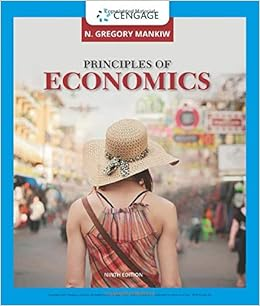The document you are viewing contains questions related to this textbook.
Chapter 15 / Exercise 3
Principles of Economics
MankiwExpert Verified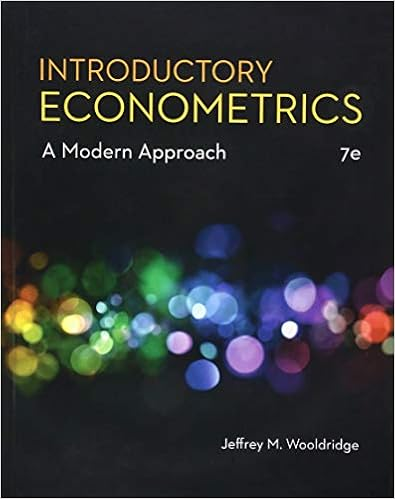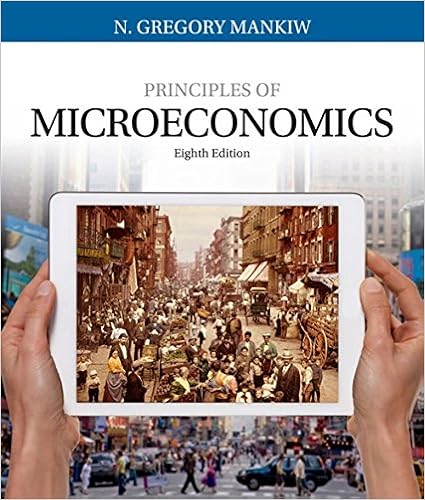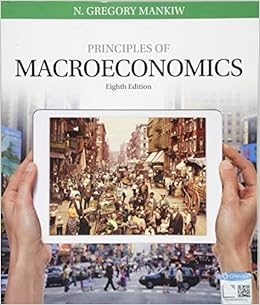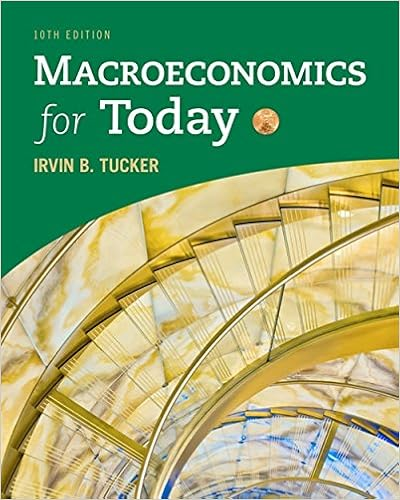Browse all Textbook Solutions
3. Limits and Continuity1. Compute the following limits:(a) limx4x2-4xx2-3x-4=45(b) limh0(4 +h)2-16h= 8(c)limx→-414+1x4 +x=-116(d) limx1x6-1x10-1=35(e) limt01t-1t2+t= 1(f) limt→-2t2-4t2+ 10t+ 16=-232. Iff(x) =x2+ 2x, compute the following limits:(a) limx2f(x)-f(1)x-1(b) limh0f(2 +h)-f(2)h(c) limh0f(a+h)-f(a)h(d) limh0f(a+h)-f(a-h)hSolution:

Course Hero member to access this document

Course Hero member to access this document

End of preview. Want to read all 62 pages?

Course Hero member to access this document

Term
Fall
Professor
staff
Tags
##### We have textbook solutions for you!
The document you are viewing contains questions related to this textbook.The document you are viewing contains questions related to this textbook.
Chapter 15 / Exercise 3
Principles of Economics
MankiwExpert Verified
•••